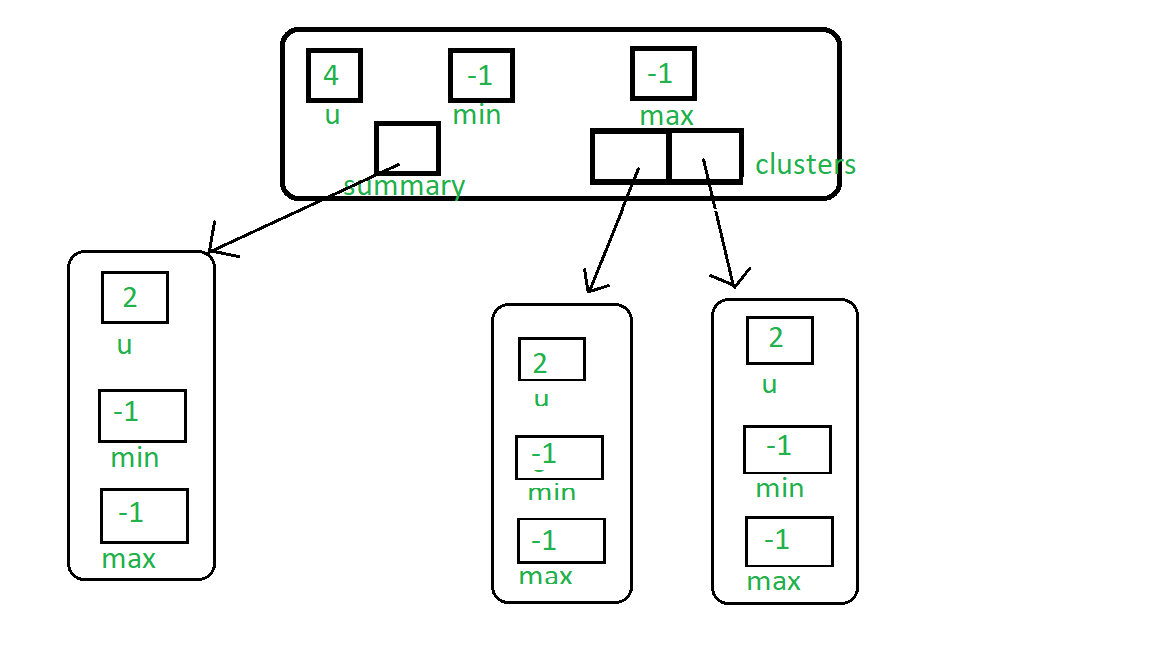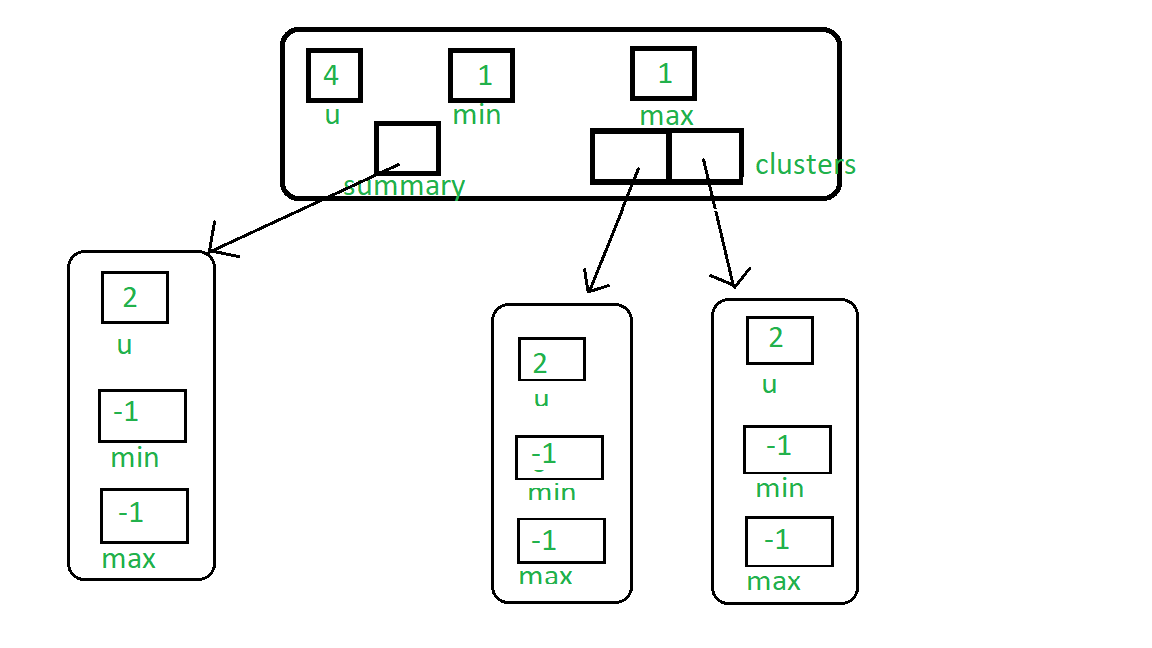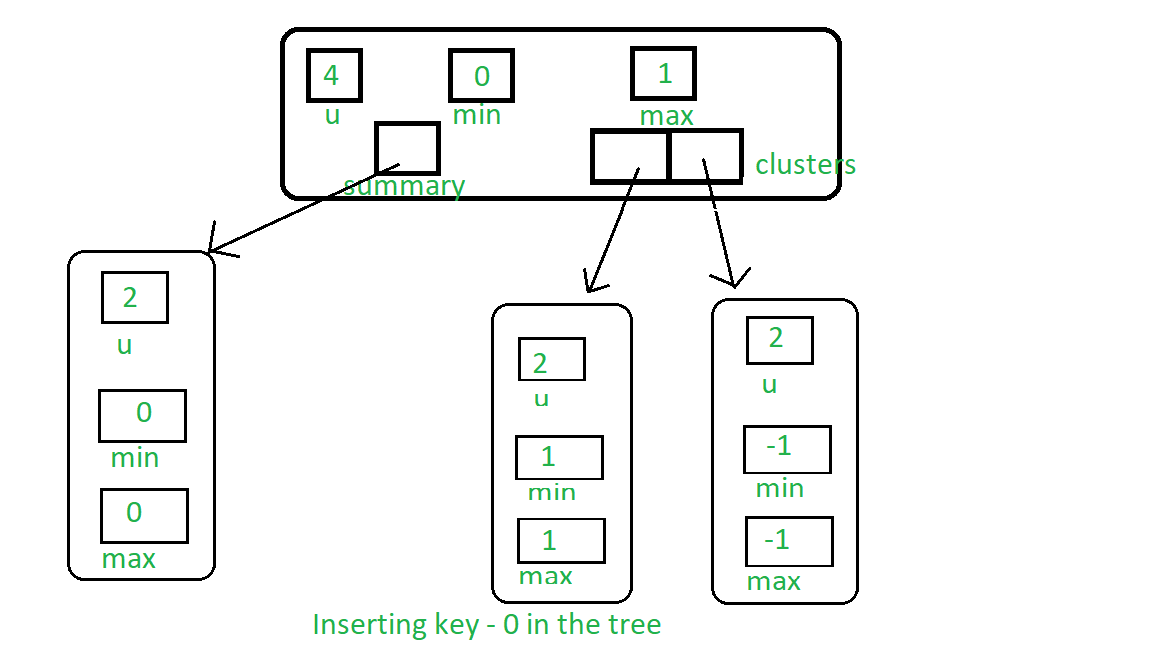# Van Emde Boas Tree | Set 2 | Insertion, Find, Minimum and Maximum Queries

It is highly recommended to see previous articles on Van Emde Boas Tree first.

Procedure for Insert :

1. If no keys are present in the tree then simply assign minimum and maximum of the tree to the key.
2. Otherwise we will go deeper in the tree and do the following:
• If the key we want to insert is less than the current minimum of the tree, then we swap both values because the new key will be the real minimum of the tree and the key which was already at the place of the minimum will be used for the further process.

This concept can be thought of as lazy propagation in Van Emde Boas Tree. Because this old minimum value really is a minimum of one of the clusters of recursive Van Emde Boas Structure. So actually we don’t go deeper into the structure until the need arises.

• If we are not at the base case means universe size of the tree is greater than 2 then :

• If the tree’s cluster[High(key)] is empty, then we recursively call insert over the summary and as we are doing lazy propagation, we just assign minimum and maximum value to the key and stop the recursion.
• Otherwise, we call insert over the cluster in which the key is present.
3. Similarly, We check for maximum and set the key as maximum if it is greater than the current maximum.

Below Image represents empty VEB(4) Tree:Now we insert 1, then it will just set the minimum and maximum of the tree to 1. You can see the Lazy propagation of 1:Now if we insert 0, then 1 will propagate to the 1st cluster and zero will be the new minimum:Procedure for isMember Query :

• At any point of our search, If the key is minimum or maximum of the tree, which means the key is present, then return true.
• If we reach the base case, but above condition is false then the key must not be present in the tree so return true.
• Otherwise recursively call the function over the cluster of the key i.e.(High(key)) and its position in the cluster i.e.(Low(key)).
• Here we are allowing universe_size to be any power of 2, so that if the situation arises in which universe_size is less than the key value then return false.

Minimum & Maximum : Van Emde Boas Tree stores minimum and maximum as its attributes, so we can return its value if it is present and null otherwise.

 `#include ` `using` `namespace` `std; ` ` `  `class` `Van_Emde_Boas { ` ` `  `public``: ` `    ``int` `universe_size; ` `    ``int` `minimum; ` `    ``int` `maximum; ` `    ``Van_Emde_Boas* summary; ` `    ``vector clusters; ` ` `  `    ``// Function to return cluster numbers ` `    ``// in which key is present ` `    ``int` `high(``int` `x) ` `    ``{ ` `        ``int` `div` `= ``ceil``(``sqrt``(universe_size)); ` `        ``return` `x / ``div``; ` `    ``} ` ` `  `    ``// Function to return position of x in cluster ` `    ``int` `low(``int` `x) ` `    ``{ ` `        ``int` `mod = ``ceil``(``sqrt``(universe_size)); ` `        ``return` `x % mod; ` `    ``} ` ` `  `    ``// Function to return the index from ` `    ``// cluster number and position ` `    ``int` `generate_index(``int` `x, ``int` `y) ` `    ``{ ` `        ``int` `ru = ``ceil``(``sqrt``(universe_size)); ` `        ``return` `x * ru + y; ` `    ``} ` ` `  `    ``// Constructor ` `    ``Van_Emde_Boas(``int` `size) ` `    ``{ ` `        ``universe_size = size; ` `        ``minimum = -1; ` `        ``maximum = -1; ` ` `  `        ``// Base case ` `        ``if` `(size <= 2) { ` `            ``summary = nullptr; ` `            ``clusters = vector(0, nullptr); ` `        ``} ` `        ``else` `{ ` `            ``int` `no_clusters = ``ceil``(``sqrt``(size)); ` ` `  `            ``// Assigning VEB(sqrt(u)) to summary ` `            ``summary = ``new` `Van_Emde_Boas(no_clusters); ` ` `  `            ``// Creating array of VEB Tree pointers of size sqrt(u) ` `            ``clusters = vector(no_clusters, nullptr); ` ` `  `            ``// Assigning VEB(sqrt(u)) to all its clusters ` `            ``for` `(``int` `i = 0; i < no_clusters; i++) { ` `                ``clusters[i] = ``new` `Van_Emde_Boas(``ceil``(``sqrt``(size))); ` `            ``} ` `        ``} ` `    ``} ` `}; ` ` `  `// Function to return the minimum value ` `// from the tree if it exists ` `int` `VEB_minimum(Van_Emde_Boas* helper) ` `{ ` `    ``return` `(helper->minimum == -1 ? -1 : helper->minimum); ` `} ` ` `  `// Function to return the maximum value ` `// from the tree if it exists ` `int` `VEB_maximum(Van_Emde_Boas* helper) ` `{ ` `    ``return` `(helper->maximum == -1 ? -1 : helper->maximum); ` `} ` ` `  `// Function to insert a key in the tree ` `void` `insert(Van_Emde_Boas* helper, ``int` `key) ` `{ ` `    ``// If no key is present in the tree ` `    ``// then set both minimum and maximum ` `    ``// to the key (Read the previous article ` `    ``// for more understanding about it) ` `    ``if` `(helper->minimum == -1) { ` `        ``helper->minimum = key; ` `        ``helper->maximum = key; ` `    ``} ` `    ``else` `{ ` `        ``if` `(key < helper->minimum) { ` ` `  `            ``// If the key is less than current minimum ` `            ``// then swap it with the current minimum ` `            ``// because this minimum is actually ` `            ``// minimum of one of the internal cluster ` `            ``// so as we go deeper into the Van Emde Boas ` `            ``// we need to take that minimum to its real position ` `            ``// This concept is similar to "Lazy Propagation" ` `            ``swap(helper->minimum, key); ` `        ``} ` ` `  `        ``// Not base case then... ` `        ``if` `(helper->universe_size > 2) { ` ` `  `            ``// If no key is present in the cluster then insert key into ` `            ``// both cluster and summary ` `            ``if` `(VEB_minimum(helper->clusters[helper->high(key)]) == -1) { ` `                ``insert(helper->summary, helper->high(key)); ` ` `  `                ``// Sets the minimum and maximum of cluster to the key ` `                ``// as no other keys are present we will stop at this level ` `                ``// we are not going deeper into the structure like ` `                ``// Lazy Propagation ` `                ``helper->clusters[helper->high(key)]->minimum = helper->low(key); ` `                ``helper->clusters[helper->high(key)]->maximum = helper->low(key); ` `            ``} ` `            ``else` `{ ` `                ``// If there are other elements in the tree then recursively ` `                ``// go deeper into the structure to set attributes accordingly ` `                ``insert(helper->clusters[helper->high(key)], helper->low(key)); ` `            ``} ` `        ``} ` ` `  `        ``// Sets the key as maximum it is greater than current maximum ` `        ``if` `(key > helper->maximum) { ` `            ``helper->maximum = key; ` `        ``} ` `    ``} ` `} ` ` `  `// Function that returns true if the ` `// key is present in the tree ` `bool` `isMember(Van_Emde_Boas* helper, ``int` `key) ` `{ ` ` `  `    ``// If universe_size is less than the key ` `    ``// then we can not search the key so returns ` `    ``// false ` `    ``if` `(helper->universe_size < key) { ` `        ``return` `false``; ` `    ``} ` ` `  `    ``// If at any point of our traversal ` `    ``// of the tree if the key is the minimum ` `    ``// or the maximum of the subtree, then ` `    ``// the key is present so returns true ` `    ``if` `(helper->minimum == key || helper->maximum == key) { ` `        ``return` `true``; ` `    ``} ` `    ``else` `{ ` ` `  `        ``// If after attending above condition, ` `        ``// if the size of the tree is 2 then ` `        ``// the present key must be ` `        ``// maximum or minimum of the tree if it ` `        ``// is not then it returns false becuase key ` `        ``// can not be present in the sub tree ` `        ``if` `(helper->universe_size == 2) { ` `            ``return` `false``; ` `        ``} ` `        ``else` `{ ` ` `  `            ``// Recursive call over the cluster ` `            ``// in which the key can be present ` `            ``// and also pass the new position of the key ` `            ``// i.e., low(key) ` `            ``return` `isMember(helper->clusters[helper->high(key)], ` `                            ``helper->low(key)); ` `        ``} ` `    ``} ` `} ` ` `  `// Driver code ` `int` `main() ` `{ ` `    ``Van_Emde_Boas* veb = ``new` `Van_Emde_Boas(8); ` ` `  `    ``// Inserting Keys ` `    ``insert(veb, 2); ` `    ``insert(veb, 3); ` `    ``insert(veb, 6); ` ` `  `    ``cout << boolalpha; ` ` `  `    ``// Checking isMember query ` `    ``cout << isMember(veb, 3) << endl; ` ` `  `    ``cout << isMember(veb, 4) << endl; ` ` `  `    ``// Maximum of VEB ` `    ``cout << VEB_maximum(veb) << endl; ` ` `  `    ``// Minimum of VEB ` `    ``cout << VEB_minimum(veb) << endl; ` `} `

Output:

```true
false
6
2
```

GeeksforGeeks has prepared a complete interview preparation course with premium videos, theory, practice problems, TA support and many more features. Please refer Placement 100 for details

My Personal Notes arrow_drop_upCheck out this Author's contributed articles.

If you like GeeksforGeeks and would like to contribute, you can also write an article using contribute.geeksforgeeks.org or mail your article to contribute@geeksforgeeks.org. See your article appearing on the GeeksforGeeks main page and help other Geeks.

Please Improve this article if you find anything incorrect by clicking on the "Improve Article" button below.

Improved By : Aakash_Panchal